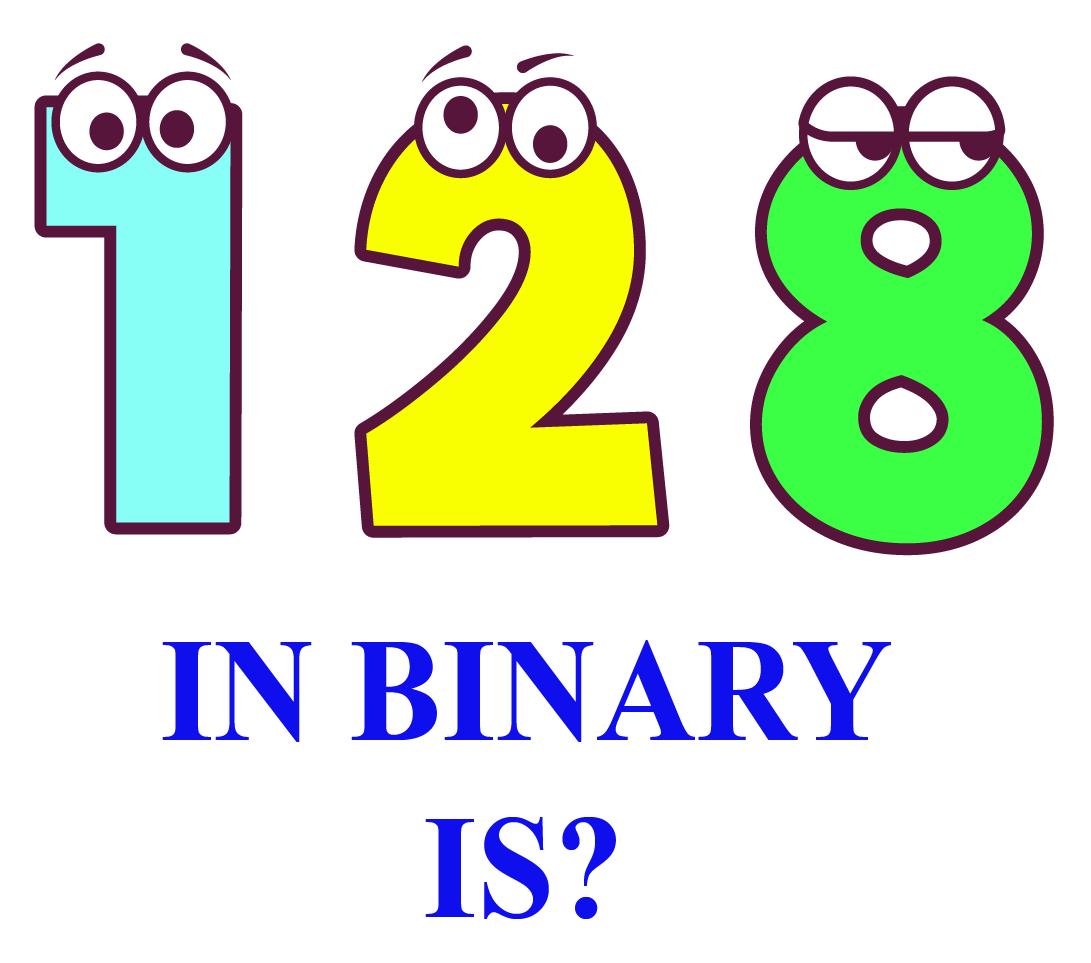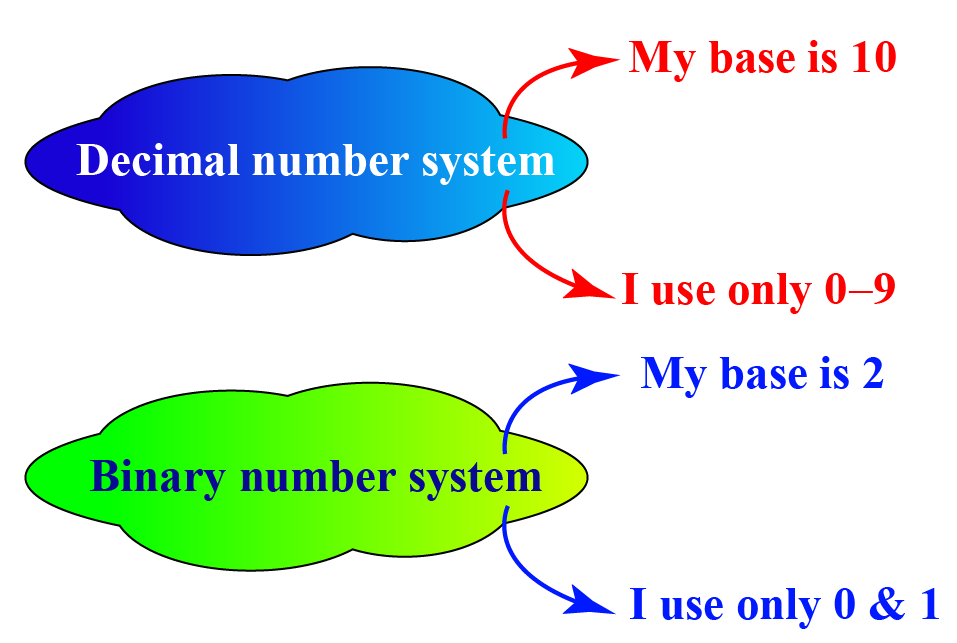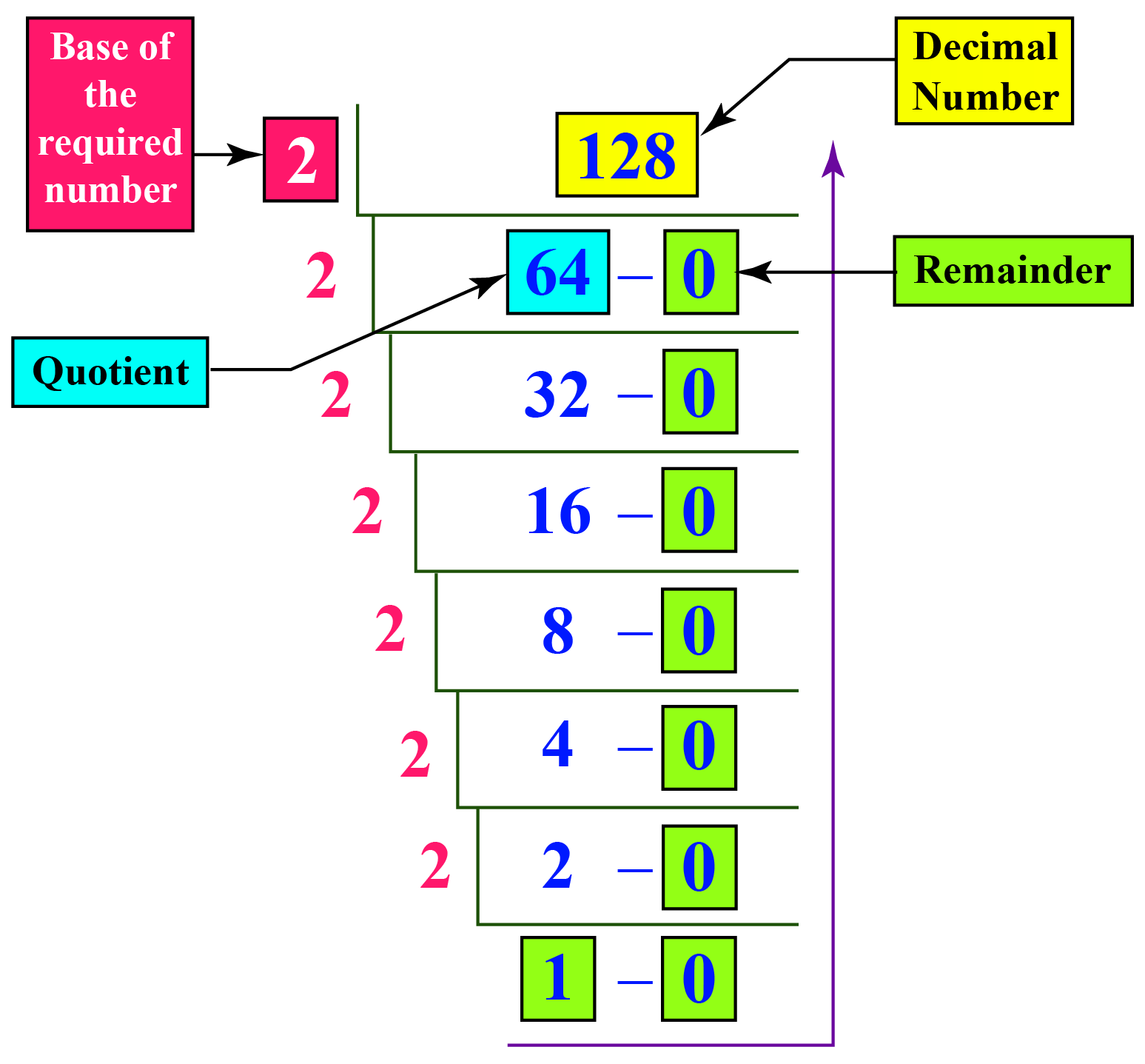# 128 in Binary

In math, we follow a standard form to represent a number. We call it the number system.

We have different types of number systems in Math.

The binary number system is one of the most common systems to be used. This system uses only two digits $$0$$ and $$1$$

Thus, the numbers in this system have a base $$2$$

$$0$$ and $$1$$ are called bits.In this mini lesson, we will explore the world of 128 in binary. We will walk through the answers to the questions like what is meant by converting 128 from decimal to binary, and how to convert 128 from decimal to binary number.

## What Is Meant By Converting 128 From Decimal to Binary?

$$128_{10}$$ is a decimal representation. Converting 128 from decimal to binary means to write or represent 128 using 2 bits only, which are 0 and 1

To convert $$128_{10}$$ in binary, we need to change the base from 10 to 2$$128_{10}$$ in binary is $$10000000_{2}$$

## How to Convert 128 From Decimal to Binary Number?

Let us look at the steps showing the conversion of 128 from decimal to binary.

Step-1

Identify the base of the required number. In this case, the base of 128 is 10, i.e., $$128_{10}$$.

Step-2

Divide the given number 128 by the base of the required number, i.e. 2

Step-3

Note down the quotient and the remainder.

Step-4

Divide the number 128 until we get the quotient to be less than the base which is 2

Step-5

Read all the remainders including the last quotient from bottom to top.

(Note: digits marked in the green boxes represent the binary form of 128.)

Repeat this process (dividing the quotient again by the base) until we get the quotient to be less than the base which is 2$$128_{10}$$ in binary is $$10000000_{2}$$

More Important Topics
Numbers
Algebra
Geometry
Measurement
Money
Data
Trigonometry
Calculus